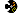CSE326 Winter 2006

 ProjectsProject 1Project 2 - phase AProject 2 - phase BProject 3 HomeworkHomework 1Homework 2Homework 3 AdministrativeHomeMessage BoardAnnoucement ArchiveClass List ArchiveAnonymous FeedbackMail Instructor & TAs LecturesCalendar & Slides HandoutsFirst Day Handout PoliciesGeneral GuidelinesGrading PoliciesProgramming GuidelinesWriteup Guidelines ComputingUnix Basics# Homework 3

## Disjoint Sets, Sorting and Graphs Due: Wednesday, March 8, beginning of class

This assignment is designed to give you practice with union-find, sorting techniques and graph algorithms discussed in the class, along with some simple applications.

Note: Please review the collaboration policy on the course web page. You must mention the names of fellow students who you worked with on this assignment.

Total: 90 points. 10 points for each problem.

1. [Union-find] Suppose you start with elements a through j with each in its own set, and perform the following operations (Showing your intermediate steps may allow partial credit):

find(d), union(d,a), union(b, c), union(h,j), find(c), union(h,b), find(j), union(b,a).
1. Without union-by-size or path compression, and choosing the root of a union by selecting the alphabetically smaller node, show the final uptree forest that results from the above operations. At what depth does node j end up in the final forest?
2. Repeat part a. but this time with union-by-size. Break any ties by giving preference to the alphabetically smaller node.
3. Repeat part b. but now add path compression as well.

1. [Sorting] Problem 7.17, page 262.  For part (c) give an average-case analysis. (runtimes for merge-sort)
2. [Sorting] Problem 7.20, page 262.  For part (c) give an average-case analysis. (runtimes for quicksort)
3. [Sorting] Sort an array containing: 3,1,4,8,5,9,2,6,7,0, using quicksort with median-of-three partitioning.  Use the code in the book for this and a cutoff value of 3 (pages 246 and 247).  Show what the recursive calls are and what the pivot and array are at the beginning of each step.  Be clear about when the cutoff kicks in and insertion sort is used.
4.  [Sorting] (5 points each part)
1. Problem 7.3, page 261 (inversions)
2. Problem 7.41, page 264 (comparison sorting 4 elements)
5. [Graphs] Suppose you are given a graph that has negative-cost edges but no negative-cost cycles. Why does the following strategy fail to find shortest paths: uniformly add a constant k to the cost of every edge so that all costs become non-negative, run Dijkstra's algorithm, return the result with edge costs reverted back to the original costs (i.e. with k subtracted). Give an argument as well as a small example where it fails.
6. [Graphs] Problem 9.1, page 341 (topological sort)
7. [Graphs] Problem 9. 5, parts (a) and (b) page 342. Be sure to give the path AND its length. (weighted and unweighted shortest path)
8. [Graphs] Problem 9. 15, parts (a) and (b) page 343. Start Prim’s algorithm with node D. (MST)